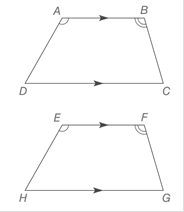Chapter 4.1, Problem 39EElementary Geometry For College St...

7th Edition
Alexander + 2 others
ISBN: 9781337614085

Solutions

Chapter
SectionElementary Geometry For College St...

7th Edition
Alexander + 2 others
ISBN: 9781337614085
Textbook Problem

In quadrilaterals ABCD and EFGH, A B - ∥ D C - , E F - ∥ H G - , ∠ A ≅ ∠ E , and ∠ B ≅ ∠ F .a) Are quadrilaterals ABCD and EFGH congruent?b) Are ∠D and ∠H congruent?To determine

a.

To find:

The quadrilaterals ABCD and EFGH congruent or not.

Explanation

Given:

In quadrilaterals ABCD and EFGH, AB-DC-, EF-HG-, AE, and BF.

Definition:

If quadrilateral ABCD is congruent to quadrilateral EFGH, then all corresponding pairs of angles (A and E ) must be congruent, as the pair of corresponding (AB- and EF-) are congruent

To determine

b.

To find:

The angles ∠D and ∠H are congruent or not.

Still sussing out bartleby?

Check out a sample textbook solution.

See a sample solution

The Solution to Your Study Problems

Bartleby provides explanations to thousands of textbook problems written by our experts, many with advanced degrees!

Get Started

Find more solutions based on key concepts